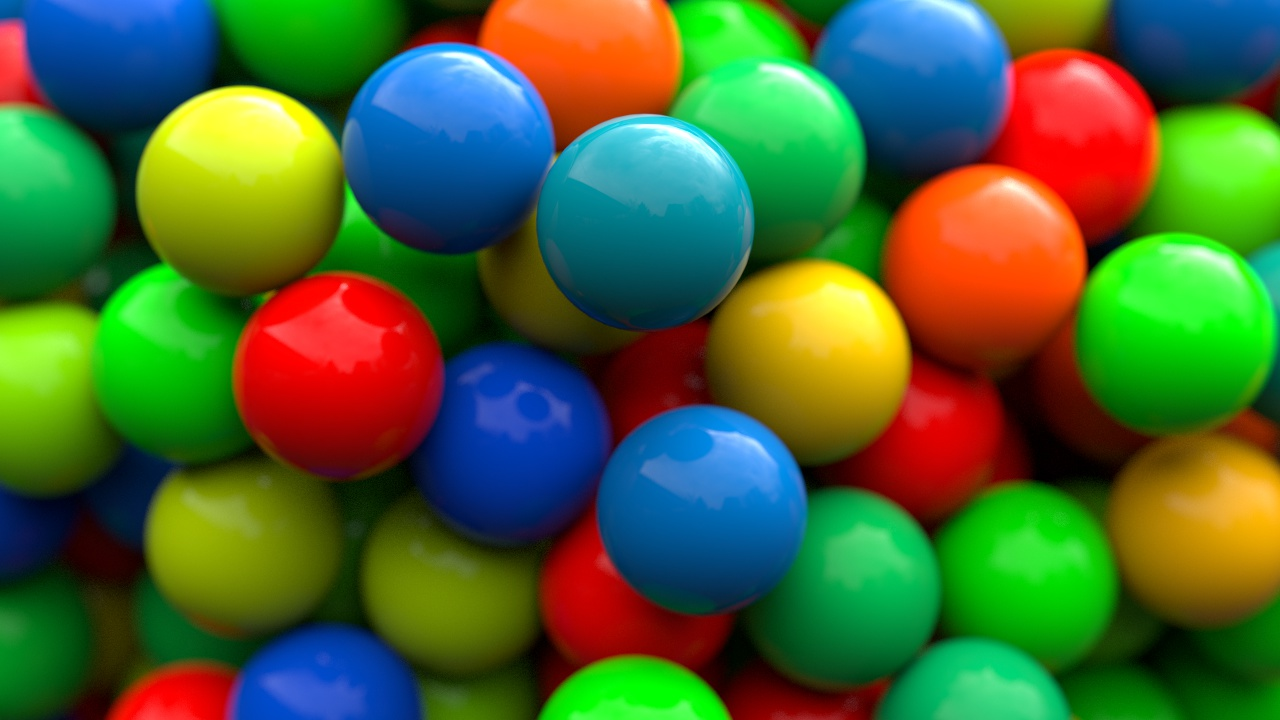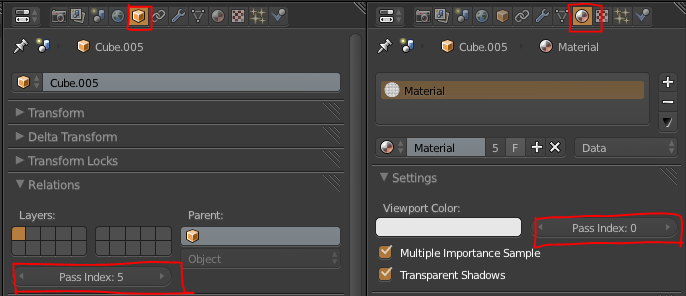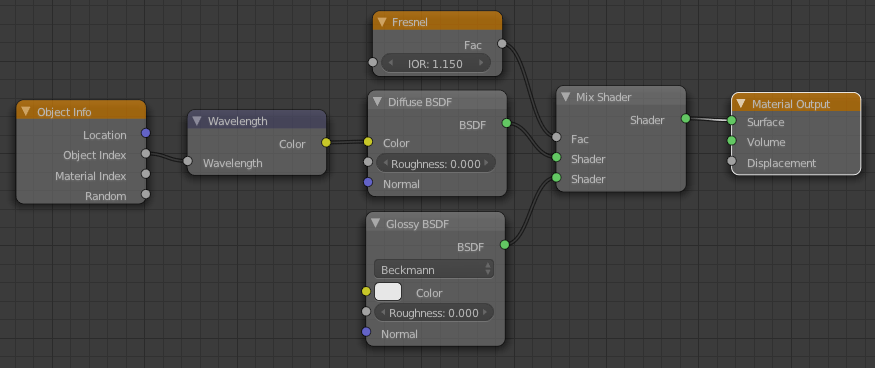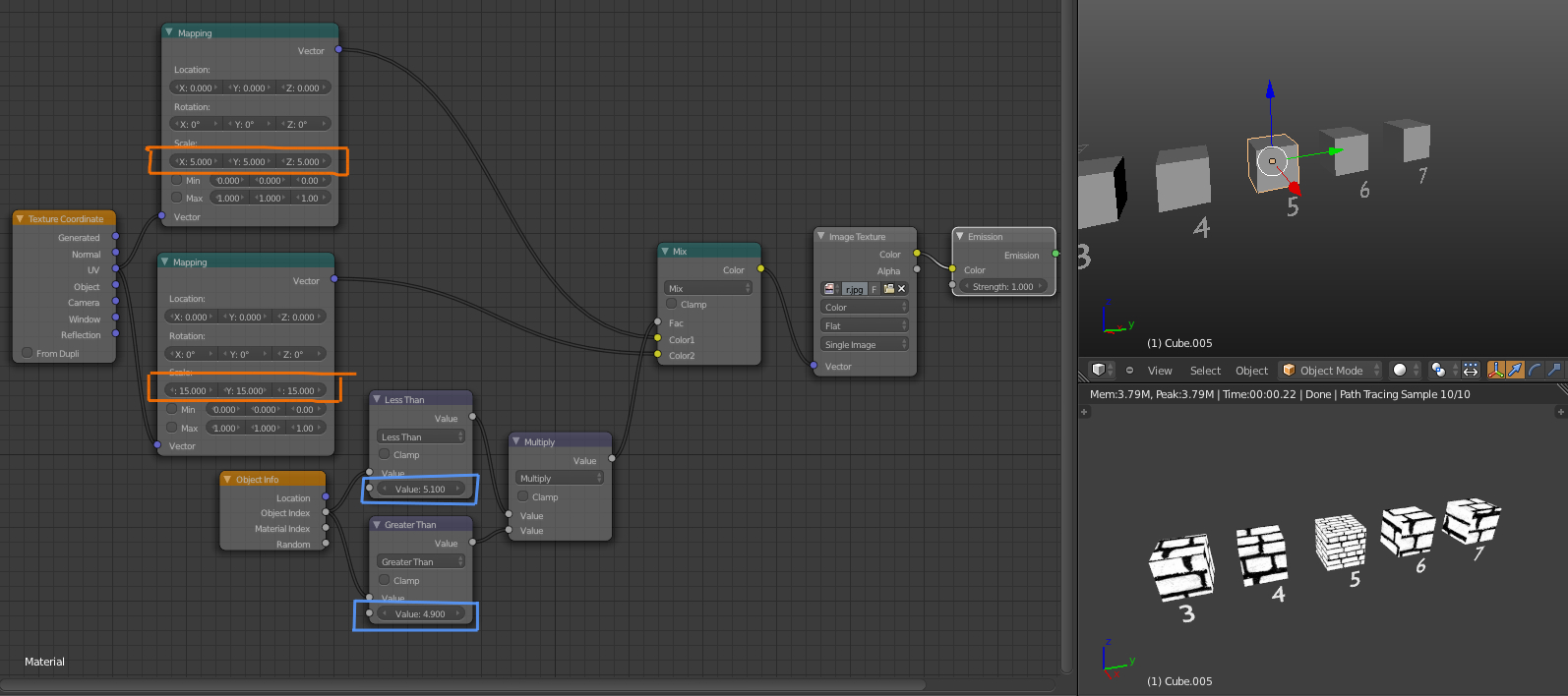Now before you ignore me, I’m not talking about compositing here. I’m talking about the Object Index output of the Object Info material node:The cool thing about this is that if you give a whole bunch of objects a single material, then you can still vary the material for each object by using the Object or Material index:In the image at the top, I used a little bit of python to give each selected object a random index inside a certain range:

import random
for obj in bpycontext.selected_objects:
obj.pass_index=int(random.random()*(640-440)+440)

The range (between 440 and 640) is actually the range of colours in wavelengths. So plugging that into the wavelength node will give me a random colour for every object:But we could do that with the Random output of the same node, albeit with less control, so here’s an even better example:

When you have a bunch of objects using the same material and you only want to change one of those objects, for example scale the texture up or down, you can use that object’s index as a mask and mix two things together with it. In the case below, making the object that has a pass index of 5 use tighter textures:Since we currently lack an “Equal To” mode for the math node, we need to use the product of Less Than and Greater Than modes. The object index is an integer, so using 5.1 and 4.9 for those nodes respectively we can be sure that we’re only getting the index of 5.0

Optimization Tip:

The mapping node’s Scale ability is really just multiplying the UVs (which is really just an ‘image’ where red and green represent X and Y coordinates, plug the UVs into an a shader’s colour if you don’t believe me). So instead of using two mapping nodes in the example above, use a Colour Mix node on Multiply with the same scaling values for the R, G and B and use the Object Index for it’s Fac.